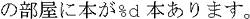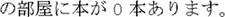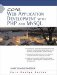# Formatted Output

Now that we have a basic understanding of locales and some of the challenges associated with using them, we can look at formatting output. We will cover the formatting of numbers and currencies and show a few helpful functions that PHP provides for the construction of strings with parameterized data.

#### Formatting Numbers

The formatted output of numeric data is most commonly done with the number_format function. This function defaults to taking two parametersthe number you wish to be formatted, and the number of decimal places to display:

` echo number_format(123345789.961, 2); `

It then formats the number according to the information for the current locale using the appropriate thousands separator and decimal character. The output for the preceding function on an American English system would be 123,345,789.96.

This function accepts more parameters that let you specify formatting characters different from those to which your current locale defaults. The optional third parameter specifies the character to use as the decimal point for fractions, and the fourth parameter is the thousands separator. (If you specify the third parameter, you must also specify the fourth.) You would use the following to print numbers as seen in France (even if your locale settings are not configured for the format):

` echo number_format(123345789.961, 2, ',', ' '); `

The output from this code would be 123 345 789,96.

#### Currencies

The formatted output of currencies in PHP can be performed with the money_format function, provided that PHP is running on a Unix-based server with the strfmon function in its C runtime library. This means that users of PHP on Microsoft Windows are unable to use this function. Given this unfortunate limitation and given that number_format does most of the necessary work, we can write our own function to perform basic currency formatting (see Listing 21-1).

##### Listing 21-1. currency_format: A Function for Formatting Monetary Data
` <?php // // somebody has to have called setlocale() before calling // this function !!! // function currency_format (   \$in_amount,            // amount to format   \$in_dec_pl = 2,        // num dec places to show   \$in_show_curr = TRUE,  // do we show the currency value?   \$in_use_symbol = TRUE  // show it as a sym (\$) or text (USD)? ) {   \$locale = localeconv();   \$formed_num = number_format(\$in_amount,                               \$in_dec_pl,                               \$locale['mon_decimal_point'],                               \$locale['mon_thousands_sep']);   if (\$in_show_curr)   {     if (\$in_use_symbol)       \$symbol = \$locale['currency_symbol'];     else       \$symbol = \$locale['int_curr_symbol'];     if (\$in_amount >= 0)     {       if (\$locale['p_cs_precedes'])       {         // put a space if we're using text currency or          // the locale says to         \$space = (!\$in_use_symbol || \$locale['p_sep_by_space'])                  ? ' ' : '';         \$formed_num = \$symbol . \$space . \$formed_num;       }       else       {         // put a space if we're using text curr (i.e. EUR)         \$space = (\$in_use_symbol) ? '' : ' ';         \$formed_num = \$formed_num . \$space . \$symbol;       }     }     else     {       if (\$locale['n_cs_precedes'])       {         \$space = (\$in_use_symbol) ? '' : ' ';         \$formed_num = \$symbol . \$space . \$formed_num;       }       else       {         \$space = (\$in_use_symbol) ? '' : ' ';         \$formed_num = \$formed_num . \$space . \$symbol;       }     }   }   return \$formed_num; } ?> `

This function accepts four parameters:

• \$in_amount The amount to format as a currency. This is a floating-point number. (See the "A Caution for Currency Data" sidebar.)

• \$in_dec_pl (default value of 2) The number of decimal places to show for the number.

• \$in_show_curr (defaults to trUE) Controls whether to show a currency symbol or format it as a number.

• \$in_use_symbol (defaults to trUE) If we decide to show the currency symbol, this controls whether or not we show the symbol (for example, \$ or ¤) or the international currency name (for example, USD or EUR).

As you look through the code in the listing, you can see that the function operates in the following manner:

• First, it gets the numeric locale information and formats the number with the appropriate number of decimal places.

• If it was instructed to display a currency symbol, it figures out if the currency symbol comes before or after the number.

• Since the locale information lets different locales specify different locations for positive and negative numbers, we must look at the number to see where the symbol goes. For example, a particular locale might choose to display CUR 1.234,56 for a positive number, but 1.234,56 CUR for a negative number.

• One complication is that some locales can insert an extra space between a symbolic currency symbol and a positive number. Thus, while one country might want \$1,234.56, another might insist upon \$ 1,234.56.

The function we have written is rather basic, but it will serve our purposes in this book.

To use this function, we can call it as follows:

` <?php   // we have to call setlocale before we can call localeconv   setlocale(LC_ALL, 'english');   // Windows   setlocale(LC_ALL, 'en_US');          // Linux   echo currency_format(1234567.89);  echo "<br/>\n";   setlocale(LC_ALL, 'italian');   // Windows   setlocale(LC_ALL, 'it');        // Linux   echo currency_format(1234567.89, 2, TRUE, FALSE); ?> `

The output of the preceding code with our formatting function would be

` \$1,234,567.89 EUR 1.234.567,89 `

## A CAUTION FOR CURRENCY DATA

As we mentioned in Chapter 9, "Designing and Creating Your Database," currency is precise data and is therefore extremely ill-suited to represent data types, such as floating-point types. Since PHP has no currency data type, currency data is almost always handed to us in code in string format when we retrieve it from a database. This may leave you wondering how you are supposed to work with currency in code and how you should pass it to functions, such as number_format or currency_ format.

The second problem is easier to solve. The data returned to us by the database server can be converted to floating-point numbers for output. For simple data presentation, the floating-point number will not introduce problems; we can be sure that it will be reliably reproduced on output:

` <?php   // we have executed a query with a mysqli obj   \$row = \$results->fetch_assoc();   // convert the number to float and pass it on   echo currency_format((float)\$row['price']); ?> `

The trickier problem is how to work with currency values in code. If we want to compute the sales tax (sometimes called a value added tax) on an item with a tax rate of 8.2 percent, we have to do some arithmetic. Avoiding the use of floating-point numbers is difficult since there are no classes to help us avoid them. More extreme solutions range from using integer numbers (and using multiplication and division to simulate decimal digits) to writing custom classes to perform currency manipulations.

#### Other Formatting Functions

A useful technique for customizing output in your program is to use parameterized strings. In this scheme, you have a template string containing placeholders that you insert data into before you send the string to output.

In PHP5, this functionality is obtained by using the sprintf function. This function takes a format string with placeholders along with parameters to insert into the placeholders. Every placeholder begins with the % character and varies according to the type of data you are inserting (see Table 21-3).

##### Table 21-3. Type Specifiers for sprintf and Friends

Type Specifier

Description

%

Prints a % character

d

Prints an integer value

f

Printed as a (locale-aware) floating-point number

s

Printed as a string

b

Prints an integer number in binary format

c

Prints an integer number as the ASCII character with that value

e

Prints a number in scientific notation (for instance, 6.02214e23)

u

Prints an integer as an unsigned integer

F

Prints a floating point number in a non-locale-aware format (for instance, U.S. English)

o

Prints an integer number in octal format

x

Prints an integer value in hexadecimal format (with letters in lowercase)

X

Prints an integer value in hexadecimal format (with letters in uppercase)

While the sprintf function is largely not multi-byte character set enabled, we can safely use it with UTF-8 strings. The only escape sequences for which it looksthose beginning with the % charactercannot form the trailing bytes of UTF-8 characters. Therefore, we can be sure that it will not process inappropriate characters. However, we will continue to be careful and verify our output frequently when we use it in localized web sites.

To demonstrate the insertion of an integer value and string value into a formatted string, we might execute the following:

` <?php   echo sprintf("There are %d books in %s's room.",                \$cbooks, \$name); ?> `

If \$cbooks was 123 and \$name was Michiko, the output from this would be

` There are 123 books in Michiko's room. `

There are a number of options we can include with this type specifier that further control how the output is generated:

• We can include a + sign before numeric type specifiers to indicate that positive numbers should have a number sign (instead of the default that only negative numbers get a sign). An example would be %+d.

• We can specify the number of decimal digits we would like for floating-point numbers by including .## before the type specifier f, where ## is the number of decimal digits we want to see. For example, %.10f would show 10 digits after the decimal place.

• We can specify a minimum width for the output data. If the output is greater than this width, it is not truncated. If the output is less than this, the output is padded from the left with spaces (by default). An example would be %10d.

• If our minimum width is greater than the width specified for the output, we can specify the character to use for padding. The default character is a space, but we can make another character by using a single quote (') and the single-byte character we wish to use. For example, to use the _ character instead of spaces, write %'_10f.

• Finally, we can specify whether the padding should be on the right or on the left if our width is too wide. This is done with a minus sign () and is specified before the width specifier: %-10f.

To see these in action, we show some examples:

` <?php \$floatv = 123456.78; \$negi = -123456; \$posi = 54829384; \$name = "Taleen"; echo sprintf("%d", \$posi);          // prints: 54829384 echo sprintf("%d", \$negi);          // prints: -123456 echo sprintf("+%d", \$posi);         // prints: +54829384 echo sprintf("0x%x", \$posi);        // prints: 0x344a148 echo sprintf("0x%X", \$posi);        // prints: 0x344A148 echo sprintf("%e", \$floatv);        // prints: 1.23457e+5 echo sprintf("%14.4f", \$floatv);    // prints: 123456.7800    ' echo sprintf("%'_15.4f", \$floatv);  // prints: ____123456.7800 echo sprintf("%-'_15.4f", \$floatv); // prints: 123456.7800____ echo sprintf("%s", \$name);          // prints: Taleen echo sprintf("'%'_12s'", \$name);    // prints: '______Taleen' ?> `

If we take another look at the first example, we would see a problem develop if we wanted to localize our application into another language, such as Japanese. The English parameter string

` "There are %d books in %s's room." `

would become, in Japanese

` "%s" `

If we were to pass this string to the same sprintf function call we made previously, as follows:

` <?php   if (\$language == "jp")     \$format = "%s";   else     \$format = "There are %d books in %s's room.";   echo sprintf(\$format, \$cbooks, \$fname); ?> `

we would get the following output in Japanese:

` 123`

Translated into English, this means: "In 123's room, there are 0 books." What happened? Japanese has very different word ordering from English (it is often perceived as "backward" to native English speakers), and we are thus obliged to reorder the type specifiers (%d and %s) in order to make the sentence work. Even though we can substitute new format strings dependent on the locale, our code is still fixed, and the parameters we pass to the sprintf function still come in the same order. Imagine our frustration then if we had to write code to account for every possible combination of orderings in parameterized strings. If we had a string with four parameters, there would be 24 possible combinations!

A better solution is provided by sprintf. This allows us to not only set a type specifier for a parameterized value, but also to indicate the parameter in which its value is to be found (This is done by putting the parameter number and \$ right after the % character, such as %3\$d). Thus, we can write code that will work as expected, as follows:

` <?php   if (\$language == "jp")     \$format = '%2\$s%1\$d';   else       \$format = 'There are %1\$d books in %2\$s's room.';   echo sprintf(\$format, 123, 'Michiko'); ?> `

If we used double quotes in any of the preceding strings, we would have to write something so that PHP would not look for a variable named \$d or \$s:

` "There are %1\\$d books in %2\\$s's room." `

We now have the ability to write fully localized and globalized web applications, regardless of word ordering.Core Web Application Development with PHP and MySQL
ISBN: 0131867164
EAN: 2147483647
Year: 2005
Pages: 255

Similar book on Amazon